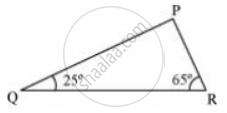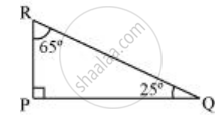Share

# Angles Q and R of a δPqr Are 25° and 65°. Write Which of the Following is True: - CBSE Class 7 - Mathematics

ConceptSum of the Lengths of Two Sides of a Triangle

#### Question

Angles Q and R of a ΔPQR are 25° and 65°.

Write which of the following is true:

(1) PQ2 + QR2= RP2

(2) PQ2 + RP2= QR2

(3) RP2 + QR2= PQ2#### Solution

The sum of the measures of all interior angles of a triangle is 180º.

∠PQR + ∠PRQ + ∠QPR = 180º

25º + 65º + ∠QPR = 180º

90º + ∠QPR = 180º

∠QPR = 180º − 90º = 90º

Therefore, Δ PQR is right-angled at point P.

Hence, (PR)2 + (PQ)2 = (QR)2Thus, (ii) is true.

Is there an error in this question or solution?

#### APPEARS IN

NCERT Solution for Mathematics for Class 7 (2018 to Current)
Chapter 6: The Triangle and its Properties
Ex. 6.50 | Q: 6 | Page no. 130
Solution Angles Q and R of a δPqr Are 25° and 65°. Write Which of the Following is True: Concept: Sum of the Lengths of Two Sides of a Triangle.
S